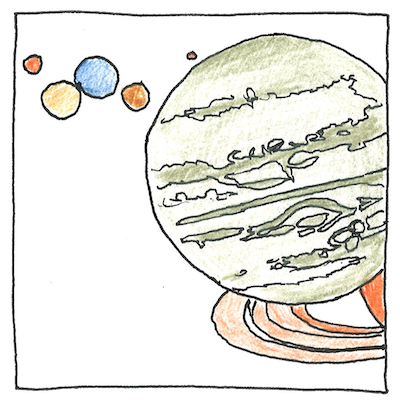# 1796

## The book of science

Tom Sharp

 Pierre-Simon Laplace astronomy

## Origin of our solar system

• The nature of spin
• helps explain how our sun
• with its planets spinning around it
• roughly in circular orbits and in the same plane
• formed from a hot mass of spinning gas.
• Laplace calculated how, as the gas contracted,
• the nebula separated from its equatorial rings
• due to turbulence and centrifugal forces,
• how each ring coalesced into a planet,
• and how heavy the sun would have to have been
• to have pulled back all its light into a black hole.

## Idealizing chaos

• Our solar system isn’t perfectly aligned.
• The planets don’t spin in perfect circles,
• their orbits aren’t exactly in the same plane,
• and comets and asteroids fly all around.
• But in mathematics we have expressed its essential principles
• and, outside of time, have rewound the system back to its beginning
• as a spinning, incandescent cloud.
• Here we discover there never was chaos except in our minds,
• only conditions unfriendly to life,
• conditions unfriendly to our arrogance,
• unfriendly to our romantic idea of progress.
• We have rolled back the universe to a point
• but we have discovered that we can compress the known
• into a remote and nested set of infinitely small homunculi
• only in our minds.

## Transforms

• Laplace taught
• that an equation expressing a function of time
• can be transformed to express
• a function of complex angular frequency
• to simplify the analysis of a system.
• If the blank page seems inviolatable, then,
• listening to your thoughts,
• begin at the upper left
• and write them down as quickly as you can
• without stopping for any reason.
• If finding a creative solution doesn’t seem likely
• then change the problem;
• change the assignment;
• change your mind;
• change your life.

Laplace was a mathematical and scientific genius. His calculation of how massive the sun would need to be for its gravity to capture all its light, removed from the second edition of Exposition du Système du Monde, was published a hundred years before Einstein’s theory of relativity.

Laplace also applied calculus to celestial mechanics, solved the “great Jupiter-Saturn inequality” and inequalities related to the movement of the moon, developed the Bayesian interpretation of probability, invented the probability-generating function, published the first statement of scientific determinism (Laplace’s demon), invented the transform of differential equations named after him, studied the equation, operator, distribution, matrix, motion, expansion, number, limit, principle (large deviations theory), and invariant named after him, built upon Thomas Young’s work on surface tension to develop the theory of capillary action (resulting in the Young-Laplace equation), and was the first to observe that the speed of sound in air depends on the heat capacity ratio.

See also in The book of science: